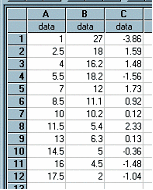﻿ ResidualX(column1, column2, regtype)

# ResidualX(column1, column2, regtype)

Top  Previous  Next
 column1 contains the independent variable's data (usually x values) column2 contains the dependent variable's data (usually y values) regtype is the regression model   ResidualX calculates the residual value of x for the y in column2 using the regtype regression model.   ResidualX operates in exactly the same manner as Residual and all three parameters are optional.  In the example, column C has been generated using ResidualX using the same data as shown for Residual.Note:  If you type decimals using a comma (eg 3,2) you should enter this formula as ResidualX(A; B; 1)  - using the ; as a separator